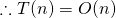# Determine a Real Number That Is Not In The List

### Problem

Given a finite set S of real numbers, design an algorithm that can output a number that is not in the list in O(n)-time.

### Solution

There are two trivial solutions that come to mind:

• Find the maximum value of the list, increment it by some positive constant and return the number.
• Sum the absolute values of the contents of the list, increment it by some positive constant and return the number.

The following algorithm is an implementation of the latter solution.

Algorithm output_not_in_list( S[0..n] )
Input: S, a list of real numbers
Output: A real number that is guaranteed to not be in the list.

begin
sum = 0;
for( i = 0 to n )
sum += |S[i]|;
}

return sum + 1;
end


### Proof of Correctness

We assume the opposite of our implication, looking for a contradiction.

Assume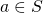and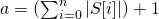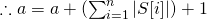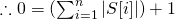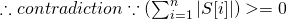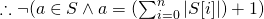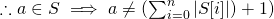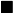### Time Complexity Analysis

The key operation involves summing the contents of the list. Since each element’s contents are accessed and added to the cumulative sum once per element, and no other non-constant functions are performed: